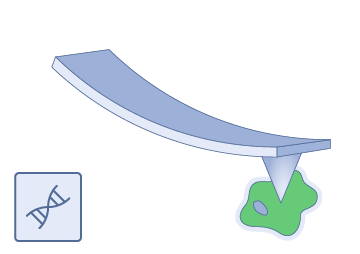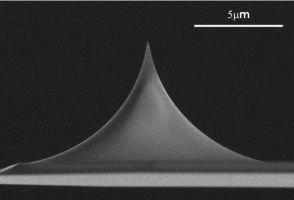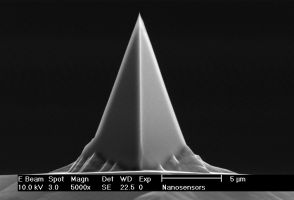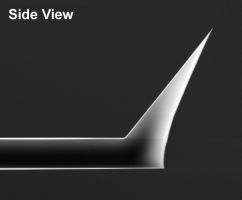## Biological Applications / Soft Contact Measurement AFM Probes### Most popular in this categoryqp-BioAC
• uniform quality SPM probe
• XY-auto alignment with Alignment Chip
• 3 cantilevers
• C1 = 0.3 N/m; fo1 = 90 kHz
• C2 = 0.1 N/m; fo2 = 50 kHz
• C3 = 0.06 N/m; fo3 = 30 kHz

### uniqprobe™qp-CONT
• uniform quality SPM probe
• XY-auto alignment with Alignment Chip
• Contact Mode
• C = 0.1 N/m; fo = 30 kHz
qp-SCONT
• uniform quality SPM probe
• XY-auto alignment with Alignment Chip
• Contact Mode
• C = 0.01 N/m; fo = 11 kHz
qp-BioT
• uniform quality SPM probe
• XY-auto alignment with Alignment Chip
• 2 cantilevers
• C1 = 0.3 N/m; fo1 = 50 kHz
• C2 = 0.08 N/m; fo2 = 20 kHz
qp-BioAC
• uniform quality SPM probe
• XY-auto alignment with Alignment Chip
• 3 cantilevers
• C1 = 0.3 N/m; fo1 = 90 kHz
• C2 = 0.1 N/m; fo2 = 50 kHz
• C3 = 0.06 N/m; fo3 = 30 kHz
qp-BioAC-CI
• uniform quality SPM probe
• special rounded tip for live cell imaging
• XY-auto alignment with Alignment Chip
• 3 cantilevers
• C1 = 0.3 N/m; fo1 = 90 kHz
• C2 = 0.1 N/m; fo2 = 50 kHz
• C3 = 0.06 N/m; fo3 = 30 kHz

### PointProbe® Plus Gold coatedPPP-NCHAuD
• Non-Contact High frequency
• Au coating on detector side
• C = 42 N/m; fo = 330 kHz
PPP-NCHAu
• Non-Contact High frequency
• Au coating (both sides)
• C = 42 N/m; fo = 330 kHz
PPP-NCLAuD
• Non-Contact Long AFM cantilever
• Au coating on detector side
• C = 48 N/m; fo = 190 kHz
PPP-NCLAu
• Non-Contact Long AFM cantilever
• Au coating (both sides)
• C = 48 N/m; fo = 190 kHz
PPP-CONTAuD
• Contact Mode
• Au coating on detector side
• C = 0.2 N/m; fo = 13 kHz
PPP-CONTAu
• Contact Mode
• Au coating (both sides)
• C = 0.2 N/m; fo = 13 kHz
PPP-FMAuD
• Force Modulation Mode
• Au coating on detector side
• C = 2.8 N/m; fo = 75 kHz
PPP-FMAu
• Force Modulation Mode
• Au coating (both sides)
• C = 2.8 N/m; fo = 75 kHz
PPP-NCSTAu
• Non-Contact Soft Tapping
• Au coating (both sides)
• C = 7.4 N/m; fo = 160 kHz
PPP-NCSTAuD
• Non-Contact Soft Tapping
• Au coating on detector side
• C = 7.4 N/m; fo = 160 kHzATEC-CONTAu
• Advanced AFM Tip at the End of the
Cantilever™
• real AFM tip visibility from top
• Contact Mode
• Au coating (both sides)
• C = 0.2 N/m; fo = 15 kHz
ATEC-NCAu
• Advanced AFM Tip at the End of the
Cantilever™
• real AFM tip visibility from top
• Non-Contact
• Au coating (both sides)
• C = 45 N/m; fo = 335 kHz
ATEC-FMAu
• Advanced AFM Tip at the End of the
Cantilever™
• real AFM tip visibility from top
• Force Modulation Mode
• Au coating (both sides)
• C = 2.8 N/m; fo = 85 kHz